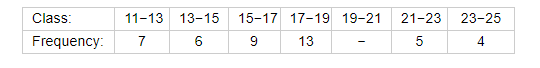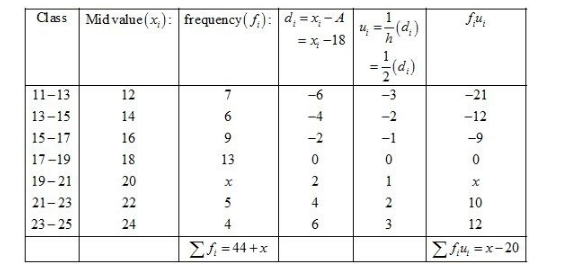# The following distribution shows the daily pocket allowance given to the children of a multistorey building.

Question:

The following distribution shows the daily pocket allowance given to the children of a multistorey building. The average pocket allowance is Rs 18.00. Find the missing frequency.Solution:

Given: Mean = 18

Suppose the missing frequency is x.

Let the assumed mean A = 18 and h = 2.We know that mean, $\bar{X}=A+h\left(\frac{1}{N} \sum f_{i} u_{i}\right)$

Now, we have $\sum f_{i}=44+x, \sum f_{i} u_{i}=x-20, h=2$ and $A=18$.

Putting the values in the above formula, we have

$18=18+2\left(\frac{x-20}{x+44}\right)$

$\Rightarrow 2\left(\frac{x-20}{x+44}\right)=0$

$\Rightarrow x-20=0$

$\Rightarrow x=20$

Thus, the missing frequency is 20.